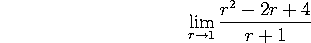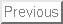This is an actual M403K midterm given on September 22, 1992. On that test I allowed a ``crib sheet'' and a non-graphing calculator.

The syllabus has changed some in 8 years, so this exam doesn't cover exactly the same material as our first midterm will. In particular, problem 4 is Chapter 2 material, which will be tested in the second midterm.

Problem 1. Tangent lines

Consider the curve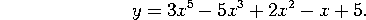a) Find dy/dx when x=1.

b) Find the slope of the line that is tangent to this curve at the point (1,4).

c) Find the equation of this line.

Problem 2. Derivatives and limits

A race car driver is circling the track at Indianapolis. The table below gives his position at various times (x denotes time, in hours. y denotes distance, in miles).

How fast is he going at time x=1?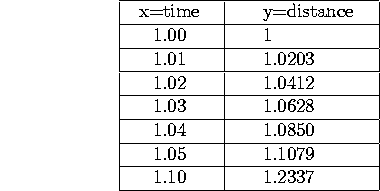Problem 3. Limits and continuity

Consider the function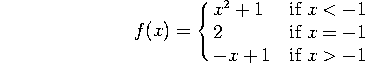a) Find the limit of f(x) as x approaches -1 from below, as x approaches -1 from above,  and the overall limit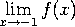, if they exist.

b) Is f(x) continuous at x=-1? Why or why not?

Problem 4. Graphs and optimization

Consider the function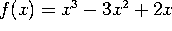.

a) Find all the partition points of f. For what values of x is f(x) positive?

b) Find all the critical points of f. For what values of x is f(x) increasing?

c) Find all the local minima of f(x).

Problem 5. Taking derivatives

Take the derivatives, with respect to x, of the following functions. You do NOT need to simplify your results:

a)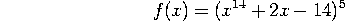b)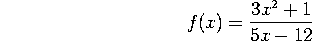c)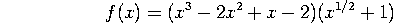d)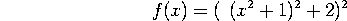Problem 6. Taking limits

Evaluate the following limits:

a)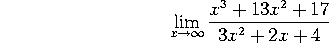b)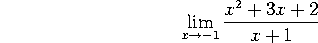c)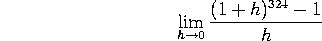d)